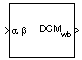# Direction Cosine Matrix Body to Wind

Convert angle of attack and sideslip angle to direction cosine matrix

• Library:
• Aerospace Blockset / Utilities / Axes Transformations

•## Description

The Direction Cosine Matrix Body to Wind block converts angle of attack and sideslip angles into a 3-by-3 direction cosine matrix (DCM). This direction cosine matrix is helpful for vector body axes to wind axes coordinate transformations. To transform the coordinates of a vector in body axes (ox0, oy0, oz0) to a vector in wind axes (ox2, oy2, oz2), multiply the block output direction cosine matrix with a vector in body axes. For information on the axis rotations for this transformation, see Algorithms.

## Ports

### Input

expand all

Angle of attack and sideslip angle, specified as a 2-by-1 vector, in radians.

Data Types: `double`

### Output

expand all

Direction cosine matrix, returned as 3-by-3 direction cosine matrix.

Data Types: `double`

## Algorithms

The order of the axis rotations required to bring this transformation about is:

1. A rotation about oy0 through the angle of attack (α) to axes (ox1, oy1, oz1)

2. A rotation about oz1 through the sideslip angle (β) to axes (ox2, oy2, oz2)

`$\begin{array}{l}\left[\begin{array}{c}o{x}_{2}\\ o{y}_{2}\\ o{z}_{2}\end{array}\right]=DC{M}_{wb}\left[\begin{array}{c}o{x}_{0}\\ o{y}_{0}\\ o{z}_{0}\end{array}\right]\\ \\ \left[\begin{array}{c}o{x}_{2}\\ o{y}_{2}\\ o{z}_{2}\end{array}\right]=\left[\begin{array}{ccc}\mathrm{cos}\beta & \mathrm{sin}\beta & 0\\ -\mathrm{sin}\beta & \mathrm{cos}\beta & 0\\ 0& 0& 1\end{array}\right]\left[\begin{array}{ccc}\mathrm{cos}\alpha & 0& \mathrm{sin}\alpha \\ 0& 1& 0\\ -\mathrm{sin}\alpha & 0& \mathrm{cos}\alpha \end{array}\right]\left[\begin{array}{c}o{x}_{0}\\ o{y}_{0}\\ o{z}_{0}\end{array}\right]\end{array}$`

Combining the two axis transformation matrices defines the following DCM.

`$DC{M}_{wb}=\left[\begin{array}{ccc}\mathrm{cos}\alpha \mathrm{cos}\beta & \mathrm{sin}\beta & \mathrm{sin}\alpha \mathrm{cos}\beta \\ -\mathrm{cos}\alpha \mathrm{sin}\beta & \mathrm{cos}\beta & -\mathrm{sin}\alpha \mathrm{sin}\beta \\ -\mathrm{sin}\alpha & 0& \mathrm{cos}\alpha \end{array}\right]$`

 Stevens, B. L., and F. L. Lewis. Aircraft Control and Simulation. Hoboken, NJ: John Wiley & Sons, 1992.